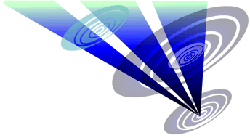Reconstruction of the 2019 Clear Wood formation 1.Draw a circle. Draw the horizontal and vertical centerlines. 2.Construct the inscribed equilateral triangle of circle 1, pointing up. 3.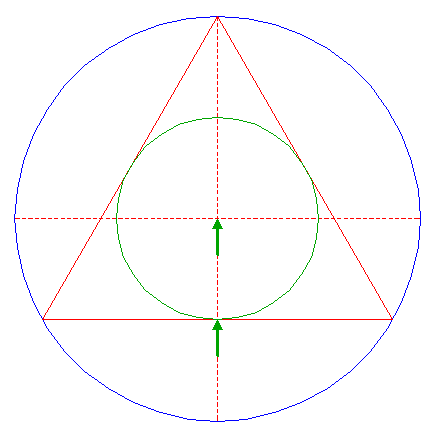Construct the inscribed circle of triangle 2. 4.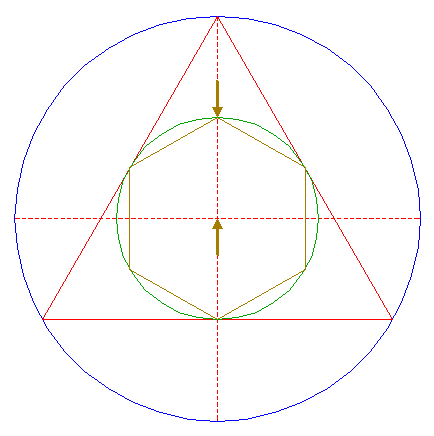Construct the inscribed hexagon (regular 6-sided polygon) of circle 3, pointing up. 5.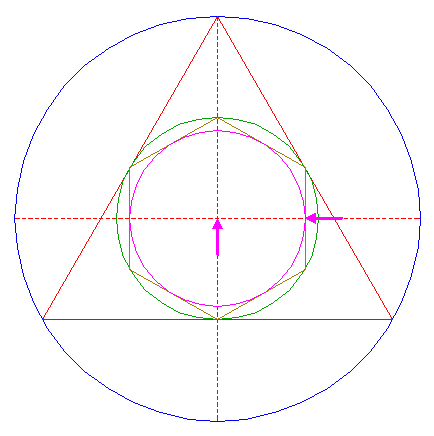Construct the inscribed circle of hexagon 4. 6.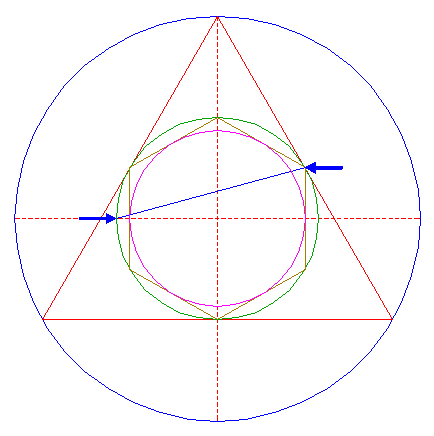Draw the connecting line between the upper righthand angular point of hexagon 4 and the lefthand intersection of circle 3 and the horizontal centerline. 7.Construct a circle concentric to circle 1, passing through the intersection of line 6 and the vertical centerline. 8.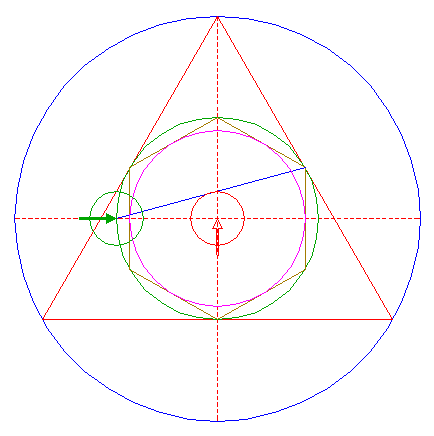Copy circle 7 to the lefthand intersection of circle 3 and the horizontal centerline. 9.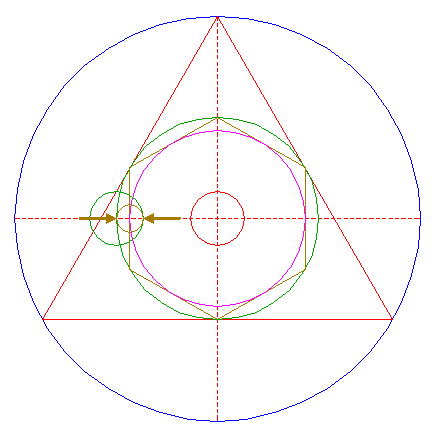Construct the "two-points" circle (defined by the two end-points of a centerline) between the center of circle 8 and its righthand intersection with the horizontal centerline. 10.Construct a circle centered at the upper intersection of circle 7 and the vertical centerline, tangent to the upper righthand side of hexagon 4. 11.Copy circle 10 to the center of circle 1. 12.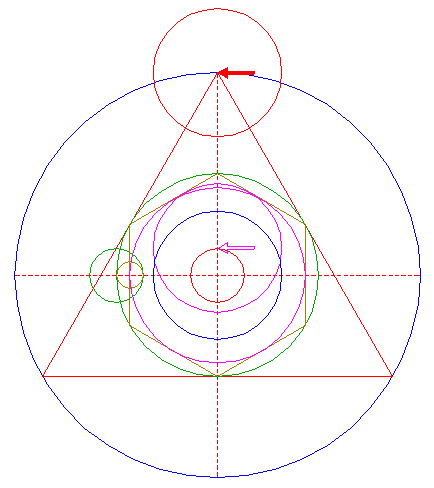Copy circle 10 to the top of triangle 2. 13.Construct a circle concentric to circle 1, passing through the intersection of circle 12 and the righthand side of triangle 2. 14.Copy circle 9 to the lefthand intersection of circle 13 and the horizontal centerline. 15.Copy circle 9 to the center of circle 10. 16.Construct a circle concentric to circle 1, tangent to circle 15 at the upper side. 17.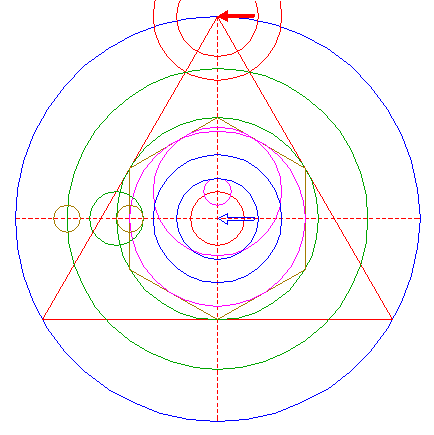Copy circle 16 to the top of triangle 2. 18.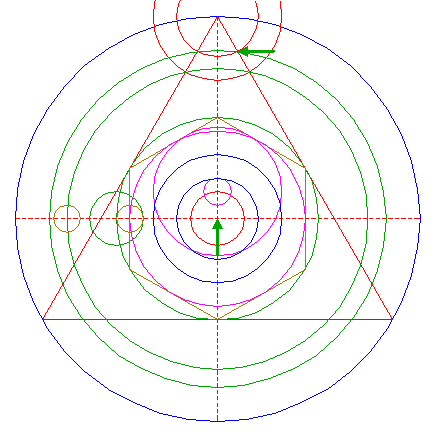Construct a circle concentric to circle 1, passing through the intersection of circle 17 and the righthand side of triangle 2. 19.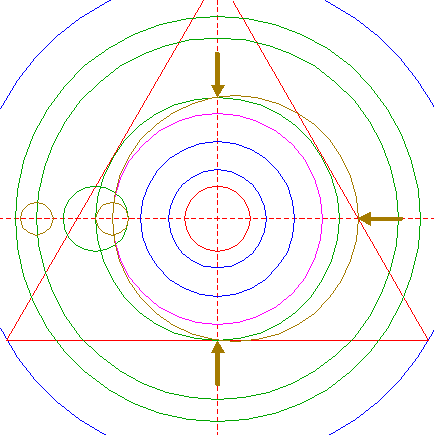Construct a circle, passing through the intersection of the righthand side of triangle 2 and the horizontal centerline, and passing through the upper and lower intersections of circle 3 and the vertical centerline. 20.Construct the "two-points" circle between the center of circle 9 and its righthand intersection with the horizontal centerline. 21.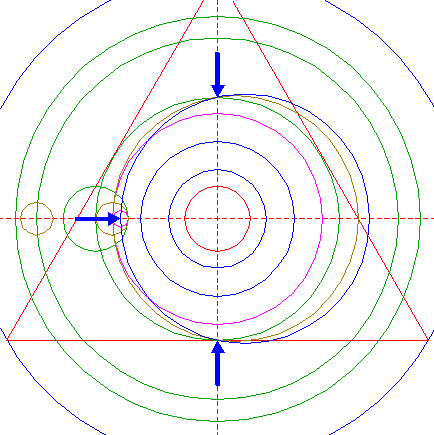Construct a circle, passing through the center of circle 20, and passing through the upper and lower intersections of circle 3 and the vertical centerline. 22.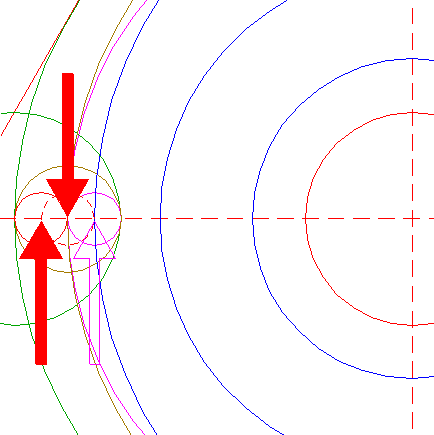Copy circle 20 to its lefthand intersection with the horizontal centerline, and move this circle (copy and delete original) to its own corresponding intersection. 23.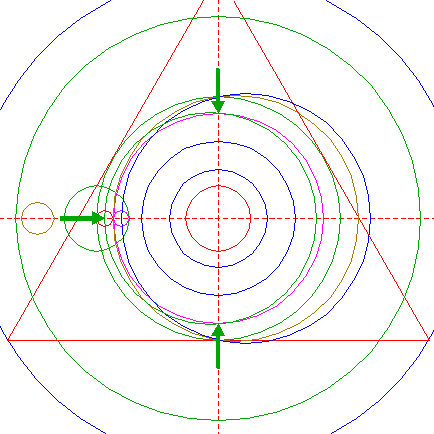Construct a circle, passing through the center of circle 22, and passing through the upper and lower intersections of circle 5 and the vertical centerline. 24.Circles 1, 5, 7, 8, 11, 14, 16, 18, 19, 21, and 23, are used for the final reconstruction. 25.Remove all parts not visible within the formation itself. 26.Colour all areas corresponding to standing... 27....or to flattened crop, and finish the reconstruction of the 2019 Clear Wood formation. 31.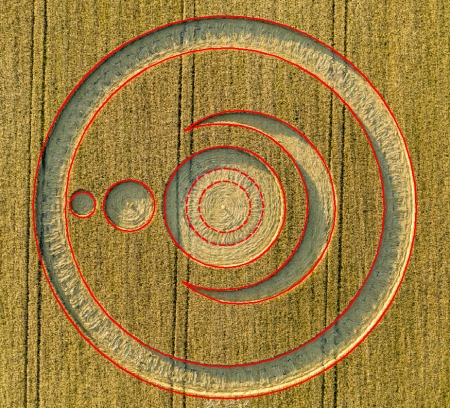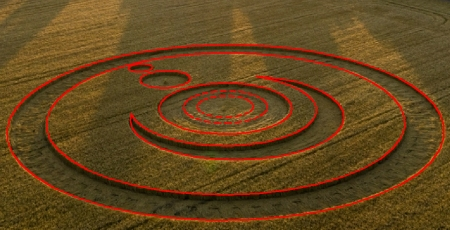The final result, matched with two aerial images.